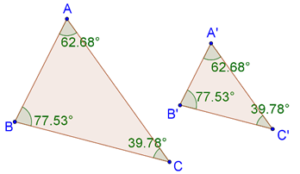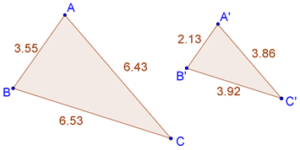# Similarity in Triangles

Go back to  'Similar-Triangles'

Now that we have understood the concept of similarity, we will start our discussion on the similarity of triangles. Two triangles are said to be similar if:

1. their corresponding angles are equal

2. their corresponding sides are in the same ratio

Consider once again the two similar triangles we encountered previously:Because they are similar, their corresponding angles are the same. Also, their corresponding sides will be in the same ratio. This means that:

$\frac{{AB}}{{A'B'}} = \frac{{BC}}{{B'C'}} = \frac{{AC}}{{A'C'}}$

Consider the following example of two similar triangles:Note that the corresponding sides are in the same proportion:

$\frac{{3.55}}{{2.13}} = \frac{{6.53}}{{3.92}} = \frac{{6.43}}{{3.86}} \approx 1.67$

The numerical values of the ratios as shown above might not be exactly the same (due to finite errors in length measurement), but in principle, these ratios must be exactly the same.

Learn math from the experts and clarify doubts instantly

• Instant doubt clearing (live one on one)
• Learn from India’s best math teachers
• Completely personalized curriculum